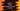# Python program to count the number of words in a file## Write a python program to count the number of words in a file :

This tutorial is to count the number of words of a file using python. We will write one python program to count the total number of words in a text file. The program will take the path of the file as an input. With this tutorial, you will learn how to open a file and read its content in python. You will also learn how to find out the list of all words from a string.

Python provides us with a lot of useful methods to work with files. We don’t need any extra module to work on any file. You can read from a file, write to a file or even append any content to a file easily using these methods. Let me quickly show you how the algorithm works :

### Algorithm :

1. Create one variable to hold the file path. This is a constant variable. In the example we are showing here, you need to change this value with the file path in your own system. Also, initialize one more variable to hold the total count of words. Initialize this variable as zero.
2. Open the file in read-only mode. We are only reading the content of the file for this example. For counting the number of words in the file, read mode will be sufficient.
3. Iterate through each line of the file using a loop. As this is a text file, we can iterate through the lines one by one.
4. Inside the loop, split the line into its words. Find out the total number of words and add them to the variable used to hold the total count of words. On each iteration of the loop, add the count of each line to this variable.
5. After the loop will complete, the word count variable will hold the total count of words in the text file. Print out the value of this variable to the user.

### Python Program :

``````word_count = 0

file_name = "D//in.txt"

with open(file_name,'r') as file:
for line in file:
word_count += len(line.split())

print ("number of words : ",word_count)``````

## Sample output:

``````The quick brown fox

jumps over the
lazy dog``````

For a file containing these words, the output will be 9.

### Explanation :

1. The program is implemented using the steps as explained in the algorithm above. ‘word_count’ is the variable used to hold the total count of all words in the text file. The value of this variable is initialized as zero. We will increment this variable by one if any word is found.
2. ‘file_name’ variable is used to hold the path of the file. Change this variable to your own file path. To find out the path of a file, you can simply drag and drop one file on the terminal. The program will not work if you don’t change this variable value.
3. We are opening the file in reading mode. The open() method is used to open a file. The first parameter of the method is the path of the file and the second parameter is the mode for opening the file. We are passing the character ‘r’, which is used to denote read-mode while opening the file.
4. Using one ‘for loop’, we are iterating through the lines of the file.
5. Inside the loop, we are splitting the line using the split() method. This method returns one list holding the words of the string. The length of this list is the word count for that line. len() method is used to find out the word count. We are adding this value to the variable word_count.
6. At the end of the program, the word_count variable holds the total word count in the file. Print its value to the user.